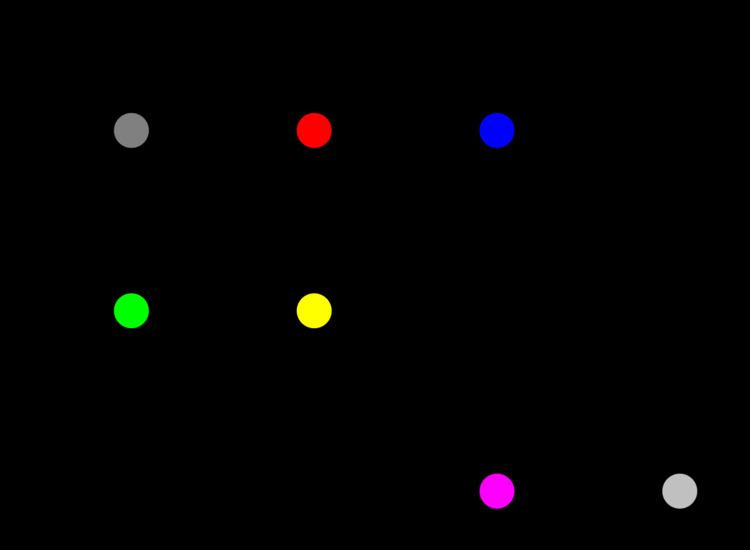# Polytope model

Updated on
Covid-19The polyhedral model (also called the polytope method) is a mathematical framework for loop nest optimization in program optimization. The polytope method treats each loop iteration within nested loops as lattice points inside mathematical objects called polytopes, performs affine transformations or more general non-affine transformations such as tiling on the polytopes, and then converts the transformed polytopes into equivalent, but optimized (depending on targeted optimization goal), loop nests through polyhedra scanning.

## Detailed example

The following C code implements a form of error-distribution dithering similar to Floyd–Steinberg dithering, but modified for pedagogical reasons. The two-dimensional array `src` contains `h` rows of `w` pixels, each pixel having a grayscale value between 0 and 255 inclusive. After the routine has finished, the output array `dst` will contain only pixels with value 0 or value 255. During the computation, each pixel's dithering error is collected by adding it back into the `src` array. (Notice that `src` and `dst` are both read and written during the computation; `src` is not read-only, and `dst` is not write-only.)

Each iteration of the inner loop modifies the values in `src[i][j]` based on the values of `src[i-1][j]`, `src[i][j-1]`, and `src[i+1][j-1]`. (The same dependencies apply to `dst[i][j]`. For the purposes of loop skewing, we can think of `src[i][j]` and `dst[i][j]` as the same element.) We can illustrate the dependencies of `src[i][j]` graphically, as in the diagram on the right.

Performing the affine transformation ( p , t ) = ( i , 2 j + i ) on the original dependency diagram gives us a new diagram, which is shown in the next image. We can then rewrite the code to loop on `p` and `t` instead of `i` and `j`, obtaining the following "skewed" routine.

Polytope model Wikipedia

Topics